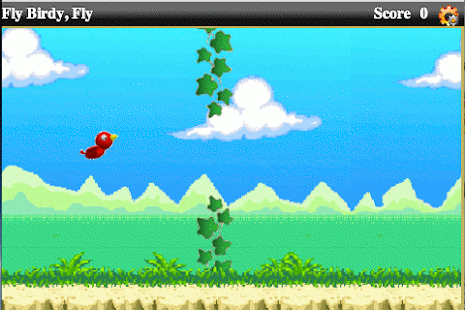## 介绍Gasp手机游戏游戏。

## 资产```<divid='board'style='position:absolute; left:50px; top:50px; width:478px;
height:300px; overflow:hidden;'><divid='score'style='position:absolute; left:400px; top:0px; height:25px;
z-index:5; color:red; font-weight:900'></div><imgclass="c"id='bird'src="b2.gif"style="z-index:5"/><imgid='instr'src='instr.png'class='c'style="left:205px; top:75px;
z-index:100"/><divclass="bg"id='bGrnd'style="top:-20px; height:320px;
background-image:url(bg1.png)"/><divclass="bg"id='fGrnd'style="top:235px; height:85px; z-index:4;
background-image:url(bg2.png)"/></div>```

## 全局变量和初始化

 鸟类 jQuery对象来支持我们的鸟类 母板 可以用于保存板对象的jQuery对象 dimPipe 障碍物尺寸 cPos 当前鸟类位置( 只有Y 坐标可以改变) 重力 可以配置的重力常数- 鸟下落的速度 iniSpeed 可以配置的初始速度 curSpeed bird的垂直速度 评分 当前得分 noClr 清除的障碍数 tmStep 用于定位鸟儿并启动障碍物的步骤计时器 状态 游戏状态：0 - 未启动，1 - 播放；2 - 游戏结束

```\$(document).ready(function() {
bird = \$('#bird');
var evt = (typeof(bird.ontouchend) == "function")
? "touchstart" : "mousedown";
board = \$('#board').bind(evt, onTap);
start();
});function start() {
state = noClr = score = 0; // not started cPos = { x: 80, y:100, h:40, w:50 };
bird.css({left:cPos.x, top:cPos.y, width:cPos.w, height:cPos.h, rotate:0});
\$('.obs').remove();
\$('#instr').show();
}```

## 点击/点击处理

```function onTap() {
if (state> 1) return;
if (state == 0) {
state = 1;
\$('#instr').hide();
tmStep = window.setInterval(BirdStep, 30);
}
curSpeed = iniSpeed;
}```

• 0 - 未运行
• 1 - 播放模式
• 2 - 模模式- 不接受输入。

```function BirdStep() {
// update bird position curSpeed += gravity;
cPos.y = Math.max(cPos.y + curSpeed, 0);
var mh = board.height()-cPos.h, m = -12, lo = 0, actPipe = \$('.obs');
bird.css({top: cPos.y});
// check if we hit the floor or other obstaclesif (cPos.y> mh)
return gameOver();
for (var i = actPipe.length-1; i> = 0; i--) {
var s = actPipe[i].style, x = parseInt(s.left), y = parseInt(s.top);
lo = Math.max(lo, x);
if (x+dimPipe.width +m <cPos.x || x> cPos.x+cPos.w+m) continue;
if (y+dimPipe.height+m <cPos.y || y> cPos.y+cPos.h+m) continue;
return gameOver();
}
// check if can launch more obstaclesif (actPipe.length> 3 || lo> 300 || Math.random()> = 0.05 * (1+noClr))
return;
var og = cPos.h * 2;
var oh = og + Math.floor(Math.random() * (mh-og+1));
var obs = \$("<img/><img/>").addClass('c obs').css({left:480, zIndex:3}).css(dimPipe).attr('src', 'vine.png')
. appendTo(board).animate({left:-50}, 3000, 'linear', function() {
\$('#score').text(' Score: ' + (score += 1 + Math.floor(++noClr/10)));
this.remove();
});
obs.style.top = oh + 'px';
obs.style.top = (oh - og - dimPipe.height) + "px";
}```

• 屏幕上已经有 4个障碍
• 最后的障碍距离一定距离
• 添加一些随机因素

## 铃声和 wistles: 视差滚动效果

```function Parallax(elm, tmo) {
elm.css('left', 0).animate({left:-15360}, {
duration:tmo*1000, easing:'linear',
complete : function() { Parallax(elm, tmo); }
});
}function onTap() {
if (state == 0) {
. . .
Parallax(\$('#bGrnd'), 240);
Parallax(\$('#fGrnd'), 80);
. . .
}
}```

## 铃声和 wistles: 旋转

```\$.cssNumber.rotate = true;
\$.cssHooks.rotate = {
set : function(el, v) {
if (typeof v === 'string')
v = (v.indexOf("rad")!= -1)? parseInt(v) * 180/Math.PI : parseInt(v);
v = (~~v);
if (v == (\$.data(el, 'rotate') || 0)) return;
el.style["MozTransform"] = el.style["MozTransform"] = el.style["-webkit-transform"]
= el.style["transform"] = " rotate(" + (v % 360) + "deg)";
\$.data(el, 'rotate', v);
},
get : function(el, computed) {
return \$.data(el, 'rotate') || 0;
}
};```

```function BirdStep() {
. . .
var ang = curSpeed * 5;
bird.css({top: cPos.y, rotate:(ang < -20)? -20 : (ang >90)? 90 : ang});
. . .
}```

```function gameOver() {
state = 2;
\$(":animated").stop();
if (tmStep) tmStep = window.clearInterval(tmStep);
bird.animate({ top:board.height()-cPos.h, rotate:540}, 1000)
. animate({ top:board.height()-cPos.h}, 500, function() {
\$('#score').text(' Score: ' + score);
start();
});
}```
that我们设置游戏状态，所以在显示动画时，我们不会检查任何点击，停止所有动画，停止小鸟计时器，等等。 之后我们可以播放'模具序列'，一旦完成后回到开始屏幕。